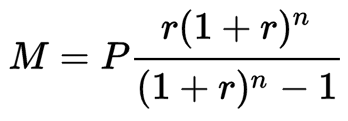# Performing Excel Functions in Cognito Forms!By Nadia A. | May 8, 2017

Cognito Forms supports robust calculations that can come in handy in a variety of scenarios - like adding a coupon code to your payment form, or conditionally hiding fields and sections. A calculation can be as simple as adding two Number fields together, or it can get quite complex; in this post, we’re going to walk through the process of using the PMT formula to calculate loan payments!

## PMT Function

In Excel, you can use the PMT function to calculate a loan payment:

`PMT(rate, nper, pv)`

The PMT function is based on the following inputs:

• Rate: The interest rate for the loan.
• Nper: The total number of payments for the loan.
• Pv: The present value, or the total amount that a series of future payments is worth now; also known as the principal.

While you do have the ability to collect this information in Cognito Forms and then export your form data to Excel, why not just use payment formula directly on your form? For example, check out this monthly mortgage payment form:

Feel free to save this demo as a template!

## Calculating PMT

To set up your own loan calculation form, you’ll need a total of five fields working together. To get started:

2. Add a Number field to your form (‘Rate’) and make sure that the field type is set to Percent.
3. Add another Number field (‘Payments’) and set the field type to Integer.
4. Add one last Number field (‘Payments Per Year’) and set the field type to Integer.
5. Lastly, add a Calculation field set to the Currency type. This is the field that will take the inputs from all the previous fields and then run them through the PMT formula. For the formula itself, use the following expression:
``````=Principal * (Rate/PaymentsPerYear) * Math.Pow(Double(1+Rate/PaymentsPerYear),Double(Payments)) / (Math.Pow(Double(1+Rate/PaymentsPerYear), Double(Payments)) - 1)
``````

So, what exactly is going on here? This expression recreates the PMT equation:Rather than using an exponent, we’re using the function Math.Pow() in order to get the correct power, as well as the function Double() in order to get the correct number to use in the power.

Have any questions about the payment function, or calculations in general? Feel free to get in touch with our support team, or just leave a comment below!